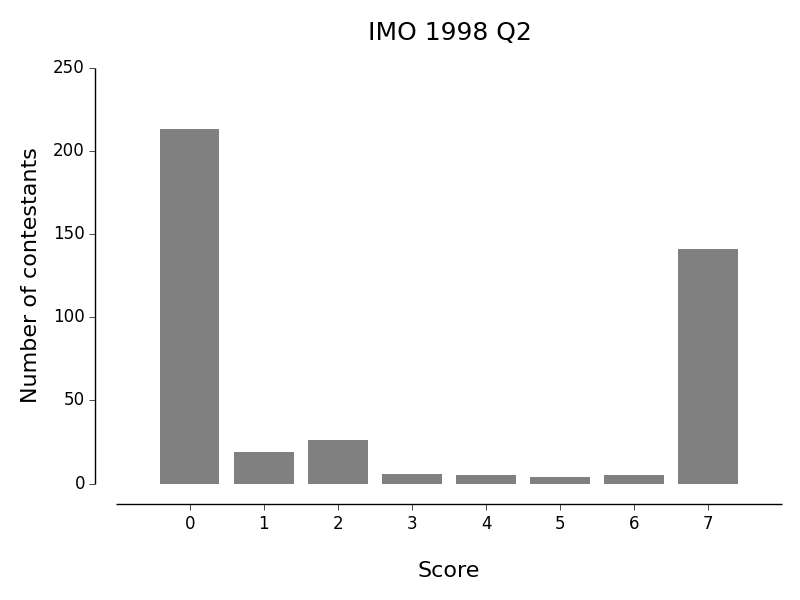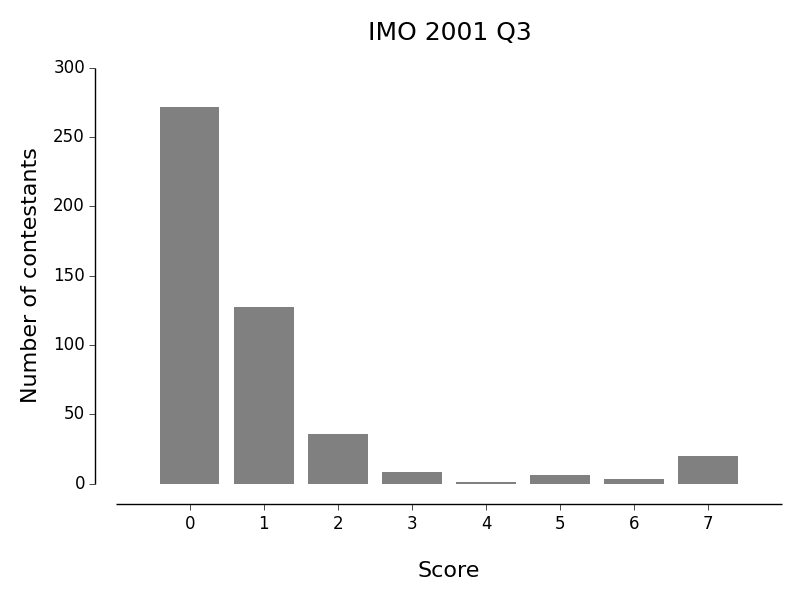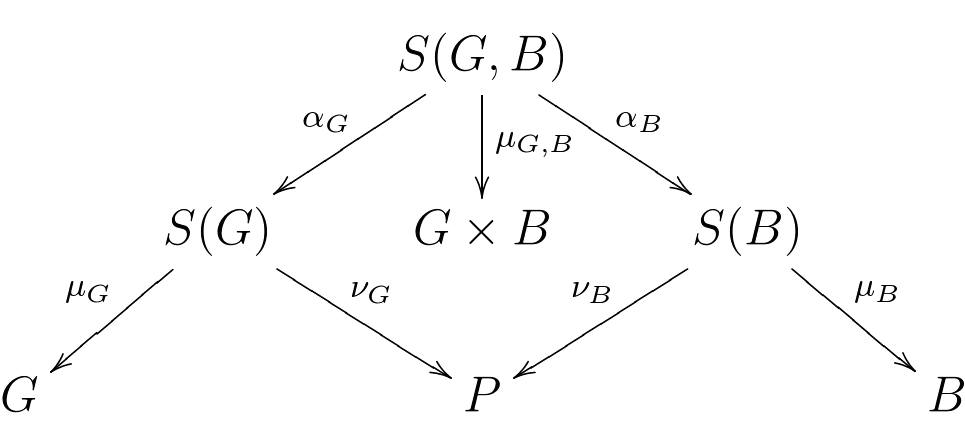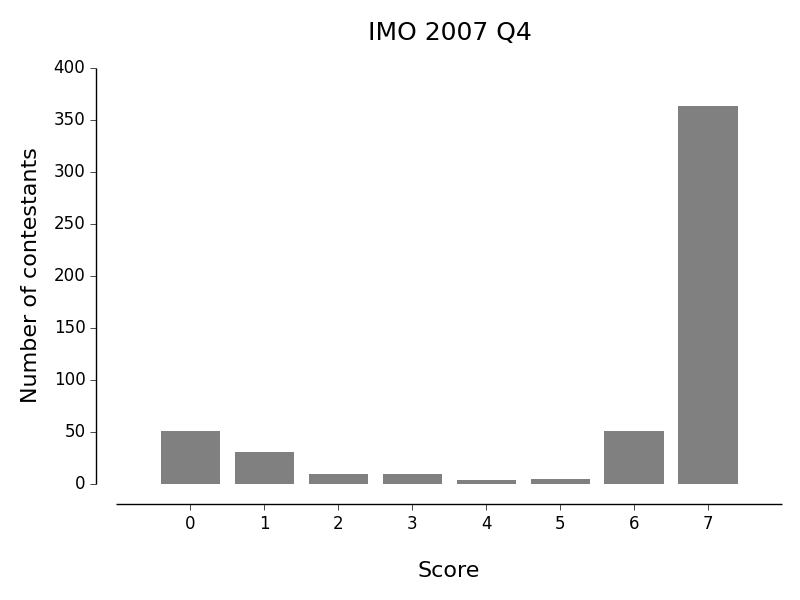## Counting what's important

### Combinatorial intuition

Some people (not me) have a gift for combinatorics that can seem almost magical at times. In other parts of mathematics I've often been surprised at how powerful a simple change in point of view can be so I wondered if I could find something simple that could contribute toward an explanation of the combinatorist's impressive skill. After all, whatever is going on, it's not actual magic.

As an experiment then, I picked some old problems from the International Mathematical Olympiad as well a simple result from finite group theory, each with what I felt had the right combinatorial flavour.

It was fun playing with these problems but in the end I didn't really feel that I had achieved my goal. On the plus side, I was a little surprised at the usefulness of one observation. It hints at the sort of thing I'd like to find so for what it's worth here it is:

When the data for a combinatorial problem can be regarded as a finite subset of a product $$S \subseteq X\times Y$$, the trivial observation that the size of $$S$$ can be regarded as the sum of the sizes its fibres over both the natural map to $$X$$ as well as the natural map to $$Y$$ can reveal apparently-tricky counting arguments to be trivial.
In other words, in terms of the diagram: $$X \xleftarrow{\mu} S \xrightarrow{\nu} Y$$ intuition sometimes fails to capture the trivial statement that: $$\sum_{x\in X}\|\mu^{-1}(x)\| = \sum_{y\in Y}\|\nu^{-1}(y)\|$$ (Note that I am not assuming $$\mu, \nu$$ are invertible; the above notation indicates set preimages.) I'll refer to this as the double-counting trick below. It is just the statement that you may count the edges of a bipartite graph grouping by either set of nodes.

### The test cases

Here are the four problems I chose:
1. IMO 1998 Q2
In a competition, there are $$a$$ contestants and $$b$$ judges, where $$b \ge 3$$ is an odd integer. Each judge rates each contestant as either "pass" or "fail". Suppose $$k$$ is a number such that, for any two judges, their ratings coincide for at most $$k$$ contestants. Prove that $$k/a \ge (b - 1)/(2b)$$.
2. IMO 2001 Q3
Twenty-one girls and twenty-one boys took part in a mathematical contest.
• Each contestant solved at most six problems.
• For each girl and each boy, at least one problem was solved by both of them.
Prove that there was a problem that was solved by at least three girls and at least three boys.
3. IMO 2005 Q6
In a mathematical competition, in which 6 problems were posed to the participants, every two of these problems were solved by more than $$\frac{2}{5}$$ of the contestants. Moreover, no contestant solved all the 6 problems. Show that there are at least 2 contestants who solved exactly 5 problems each.
4. Burnside-Polya counting lemma
Suppose that a finite group $$G$$ acts on a finite set $$X$$ then: $$\|X/G\| = \frac{1}{\|G\|}\sum_{g\in G}\|Fix(g)\|$$ where $$Fix(g) = \{x\in X~|~g\cdot x = x\}$$.
Here are the results for the first two problems (see this post for more data):Of the 419 contestants set the 1998 problem, 51% of them (including me) scored 0. For the 2001 problem, 58% of 473 contestants scored 0 and only 4% of contestants got the question fully correct. The interesting thing is that these problems are not at all deep and yet they're hard. I believe this makes them good candidates for my aim: if there's almost nothing going on mathematically there is little to obscure my hoped-for insights into how the solutions are obtained. The polarizing nature of the 1998 problem seems especially convenient: such a sharp division into two groups of candidates suggests there is a trick / natural intuition available to some but not to all.

So those are the test cases. If you like this sort of thing and are lucky enough to be possessed of 20 minutes as well as extraordinary will power, I'd suggest resisting the temptation to read on before spending about 10 minutes on each of the first two.

### Test case 1 (IMO 1998 Q2)

The problem asks us to think about triples consisting of a contestant and two judges whose ratings agree for that contestant. So we should consider the subset $$A \subseteq C\times J^{(2)}$$ of all such incidences of agreement, where $$C$$ and $$J$$ are the sets of judges and contestants and $$J^{(2)} = J\times J - \{(j,j)\}$$. We have natural maps: $$C \xleftarrow{\mu} A \xrightarrow{\nu} J^{(2)}$$ We now do the "obvious" thing and count the elements of $$A$$ in two ways: as the sum of the sizes of fibres, firstly of $$\nu$$, and secondly of $$\mu$$. Since $$\|\nu^{-1}(j,j')\| \le k$$ for all $$(j,j') \in J^{(2)}$$ and $$\|J^{(2)}\| = b(b-1)$$ we have an upper bound on the size of $$A$$: $$\|A\|\le kb(b-1)$$ On the other hand we also have a lower bound: $$\|A\| \ge a\min_{c\in C}\|\mu^{-1}(c)\|$$ So that just leaves us to consider $$\min\|\mu^{-1}(c)\|$$. Minimum agreement for a contestant occurs when the judges' ratings are split as evenly as possible. Since $$b$$ is odd this occurs when they are divided into groups of size $$(b-1)/2$$ and $$(b+1)/2$$ from which (remembering that we're dealing with ordered paris of jduges) we get: $$\|\mu^{-1}(c)\| \ge (b - 1)^2/2$$. Rearranging gives the result.

The point is, that was all totally mechanical. Now look back at the histogram of results!

Incidentally there's a pleasing interpretation of this result in terms of graph theory. We take the complete bipartite graph whose two sets of nodes are contestants and judges and colour an edge white or black according to whether the judge rated a contestant pass or fail.Using dashed lines to indicate white edges.

The argument above just counts the total number of length-two monochromatic paths from one judge to another and the statistic $$k$$ associated to such a graph is the maximum number of such paths taken over all pairs of judges. (This even suggests fun interpretations of the result, e.g., bounding the minimum number of routes between pairs of nodes wishing to communicate over a common protcol.)

### Test case 2 (IMO 2001 Q3)

It will make things clearer not to work with specific numbers so let's take the following restatement:
$$a$$ girls and $$a$$ boys took part in a mathematical contest.
• Each contestant solved at most $$n$$ problems ($$n > 1$$).
• For each girl and each boy, at least one problem was solved by both of them.
Prove there was a problem that was solved by $$x$$ boys and $$x$$ girls, where $$x = \left\lceil\frac{a}{2(n-1)}\right\rceil$$.

Let $$G, B, P$$ be the sets of girls, boys and problems and let: $$P(l, G) = \{p \in P ~|~ \mbox{at least l girls solved p}\}$$ and similarly for $$P(l, B)$$. The problem asks us to establish a lower bound for: $$k = \max\{l \in \mathbb{N} ~|~ P(l, G) \cap P(l, B) \ne \emptyset \}$$

The data supplied in the problem is a subset $$S \subseteq (G\sqcup B)\times P$$. Let $$S(G, B) = \{ (g, b, p) \in G\times B\times P ~|~ (g, p) \in S, (b, p) \in S\}$$ and note that there are natural maps: $$G\times B \xleftarrow{\mu_{G,B}} S(G, B) \xrightarrow{\nu_{G,B}} P$$ Similarly define $$S(G) \subseteq G\times P$$ and $$S(B) \subseteq B\times P$$ and note that these all fit into the commutative diagram:where $$\alpha_G, \alpha_B$$ are the obvious maps and satisfy: $$\nu_{G,B} = \nu_G\circ\alpha_G = \nu_B\circ\alpha_B$$ This diagram, which is only a bookkeeping device, was how I started the problem; I'd argue that it is natural because the constraints are now expressed as:
• The fibres of $$\mu_G$$ and $$\mu_B$$ contain at most $$n$$ points.
• The fibres of $$\mu_{G,B}$$ contain at least one point (i.e., the map is surjective).
and:
• $$P(l, G) = \{p \in P ~|~ \|\nu_G^{-1}(p)\| \ge l\}$$ (and similarly $$P(l, B)$$)

We've done nothing so far, so how should we begin? We use the double-counting trick for $$S(G, B)$$ of course! Because $$\mu_{G,B}$$ is surjective we have: $$a^2 \le \|S(G, B)\| = \sum_{p\in P}\|\nu_{G,B}^{-1}(p)\|$$ Bearing in mind the definition of $$k$$, it is natural to split this sum up into the fibres over $$P(k+1, G)$$ and its complement and to use the two different factorisations of $$\nu_{G,B}$$ to get: \begin{align} a^2 &\le \sum_{p\in P(k+1, G)}\|\alpha_G^{-1}(\nu_G^{-1}(p))\| + \sum_{p \notin P(k+1, G)}\|\alpha_B^{-1}(\nu_B^{-1}(p))\|\\ &\le k\sum_{p\in P(k+1, G)}\|\nu_G^{-1}(p)\| + k\sum_{p \notin P(k+1, G)}\|\nu_B^{-1}(p)\| \end{align} by definition of $$k$$ for the first term and by definition of $$P(k+1, G)$$ for the second. So we have: $$a^2 \le k\left(\|\nu_G^{-1}(P(k+1, G))\| + \|\nu_B^{-1}(P(k+1, G)')\|\right)$$ OK that was easy but how do we deal with these terms? We just use the double-counting trick twice more of course: once each for $$S(G)$$ and $$S(B)$$. For $$S(G)$$ it yields: $$\|\nu_G^{-1}(P(k+1, G))\| + \|\nu_G^{-1}(P(k+1, G)')\| = \|S(G)\| \le na$$ Obviously we can only make progress if we can say something about $$\nu_G^{-1}(P(k+1, G)')$$.

As far as I can see, this is the one point at which we actually need to think. Focus attention on one girl. She solved at most $$n$$ problems and yet solved at least one problem in common with each of the $$a$$ boys. By the pigeon hole principle, one of the problems she solved was solved by at least $$\lceil a/n\rceil$$ boys. In other words: $$\mu_G(\nu_G^{-1}(P(\lceil a/n\rceil, B))) = G$$ and so: $$\|\nu_G^{-1}(P(\lceil a/n\rceil, B))\| \ge \|G\| = a$$ Since $$\lceil a/n\rceil \ge \lceil a/(2(n-1))\rceil$$ we can assume $$k < \lceil a/n\rceil$$ without loss of generality and so $$P(k+1, G) \cap P(\lceil a/n\rceil, B) = \emptyset$$ by definition of $$k$$, or in other words $$P(\lceil a/n\rceil, B) \subseteq P(k+1, G)'$$. Thus by our pigeon-hole-principle result above we get: $$\|\nu_G^{-1}(P(k+1, G)')\| \ge a$$ and so combining this with the result of our double-counting argument for $$S(G)$$ we have: $$\|\nu_G^{-1}(P(k+1, G))\| \le (n-1)a$$ A similar argument for $$S(B)$$ yields: $$\|\nu_B^{-1}(P(k+1, G)')\| \le (n-1)a$$ Combining results we thus have: $$a^2 \le 2k(n-1)a$$ and the problem is solved.

Like its predecessor, there is a natural graph-theoretic interpretation of this problem; we take a graph with three sets of nodes: boys, girls, and problems with an edge joining each contestant to the problems they solved.For each problem we define the min-degree to be the minimum of the number of girls and the number of boys to which it is directly connected. The graph's statistic $$k$$ is then the maximum of min-degree, taken over all problems. In the solution above we count the total number of length-two paths between a girl and a boy, summed over all such pairs.

Incidentally the above proof generalises easily to the case that there are unequal numbers of girls and boys. For example if there are $$g$$ girls, each of whom solved at most $$n$$ problems and $$b$$ boys each of whom solved at most $$m$$ problems and if $$b+g \le \min(ng, mb)$$ then there must exist a problem solved by at least $$x$$ girls and $$x$$ boys where: $$x = \left\lceil\frac{gb}{(n-1)g + (m-1)b}\right\rceil.$$ Update 2019-07-06: see here for a much simpler solution to this problem.

### Test case 3 (IMO 2005 Q6)

This problem is strikingly similar to 1998 Q2 but trickier. It happens to concern an opposite sort of bound, but also amounts to counting length-2 paths in a bipartite graph.

Let $$C$$ be the set of contestants and $$P$$ the set of problems. Because of the $$\frac{2}{5}$$ constraint, we want counts involving problem pairs. Thus let $$P^{(2)} = \{ \{p, q\} ~|~ p, q \in P, p \ne q \}$$ be the 15-element set of unordered problem pairs.

The data of who solved which problem pairs gives us a subset $$S \subseteq C\times P^{(2)}$$ and thus natural maps: $$C \xleftarrow{\mu} S \xrightarrow{\nu} P^{(2)}$$ We mechanically apply the double counting technique. From the map $$\mu$$ we obtain $$\|S\| = C_2 + 3C_3 + 6C_4 + 10C_5,$$ where $$C_i$$ is the number of contestants who solved exactly $$i$$ problems (and we have used the fact that $$C_6 = 0$$). From the map $$\nu$$ we obtain \begin{align} \|S\| &= \sum_{x\in P^{(2)}} \|\nu^{-1}(x)\|\\ &\ge \|P^{(2)}\|\min_{x\in P^{(2)}} \|\nu^{-1}(x)\|\\ &= 15\min_{x\in P^{(2)}} \|\nu^{-1}(x)\|. \end{align} We're given: $$\min_{x\in P^{(2)}} \|\nu^{-1}(x)\| > \frac{2}{5}\|C\|,$$ and so because of integrality: $$\min_{x\in P^{(2)}} \|\nu^{-1}(x)\| \ge \left\lfloor\frac{2}{5}\|C\|\right\rfloor + 1 \ge \frac{2\|C\| + 1}{5}.$$ The double-counting trick thus yields: $$C_2 + 3C_3 + 6C_4 + 10C_5 \ge 15\cdot\frac{2(C_1 + C_2 + C_3 + C_4 + C_5) + 1}{5}.$$ This rearranges to: $$4C_5 \ge 6C_1 + 5C_2 + 3C_3 + 3,$$ which requires $$C_5 \ge 1$$. We proceed by assuming $$C_5 = 1$$ and obtaining a contradiction. By the above inequality we necessarily have $$C_1 = C_2 = C_3 = 0$$. So we are considering the case that all contestants solved 4 problems except for one who solved 5 problems, and who we will denote by: $$\hat c \in C.$$

Further, we will denote the sole problem that $$\hat c$$ did not solve by: $$\hat p \in P.$$

Now we must ask ourselves where have we been inefficient in terms of putting the data of the question to use. There are exactly two places:

1. We replaced $$\sum_{x\in P^{(2)}} \|\nu^{-1}(x)\|$$ with $$\|P^{(2)}\|\min_{x\in P^{(2)}} \|\nu^{-1}(x)\|$$.
2. When working with the map $$\nu$$, we ignored the fact that $$S$$ is constrained by the fact that it is derived from an incidence relation in $$C\times P$$.
Consider inefficiency 1; because the left and right hand sides of our double-counting inequality above differ by 1, it tells us that: $$\sum_{x\in P^{(2)}} \|\nu^{-1}(x)\| = \|P^{(2)}\|\min_{x\in P^{(2)}} \|\nu^{-1}(x)\| + 1$$ If we let $$M = \min_{x\in P^{(2)}} \|\nu^{-1}(x)\|$$, then the above means that there is a distinguished problem pair $$\hat x \in P^{(2)}$$ such that: $$\|\nu^{-1}(x)\| = \begin{cases} M + 1 & \mbox{ if } x = \hat x.\\ M & \mbox{ if } x \ne \hat x,\\ \end{cases}$$ We add this form of $$\nu$$ to our hypotheses and so have only the inefficiency 2 left to exploit.

Considering inefficiency 2, note that $$P^{(2)}$$ carries some additional structure. Specifically, it has 6 distinguished 5-element subsets, one for each $$p \in P$$: $$Q_p = \{ \{p, q\} ~|~ q\in P, q \ne p \} \subset P^{(2)}$$

A natural way to put this extra structure to work is to study the behaviour of: $$f(p) = \sum_{x \in Q_p}\|\nu^{-1}(x)\|.$$ Our hypotheses make it easy to evaluate $$f$$ in two different ways: the double-counting trick again of course! On the one hand we have: $$f(p) = \begin{cases} 5M + 1 & \mbox{ if } \hat x \in Q_p,\\ 5M & \mbox{ if } \hat x \not\in Q_p,\\ \end{cases}$$ because each problem pair in the 5-element set $$Q_p$$ contributes $$M$$ to the sum defining $$f(p)$$, except for $$\hat x$$ which contributes $$M+1$$. On the other hand we have: $$f(p) = \begin{cases} 3a_p + 1 & \mbox{ if } p \neq \hat p,\\ 3a_p & \mbox{ if } p = \hat p, \end{cases}$$ where $$a_p$$ is the number of contestants who solved problem $$p$$, because each contestant who solved $$p$$ contributes 3 to the sum except for $$\hat c$$, who contributes 4.

We have essentially arrived at the contradiction: $$f$$ is overdetermined. Choose $$p, q \in P$$ such that:

• Neither of $$p, q$$ are $$\hat p$$,
• $$p \in \hat x$$ (this is tautologically equivalent to $$\hat x \in Q_p$$),
• $$q \not\in \hat x$$.
We thus have: \begin{align} f(p) &= f(q) + 1,\\ f(p) &\equiv f(q) \pmod 3,\\ \end{align} which is a contradiction.

The above is of course just one possible solution. Prior to posting the above, I discussed this question with Ben North and he came up with a beautiful, different solution which I sketch below (actually I've slightly modified his solution but I believe I have retained its essence; all mistakes mine etc.). He begins similarly and also establishes the result by obtaining a contradiction under the hypothesis that all contestants solved 4 problems except for one who solved 5. The key difference in Ben's solution is that he double counts, not just over $$Q_p$$, but also over its complement in $$P^{(2)}$$. This provides both upper and lower bounds for $$a_p$$ as follows: $$3a_p - 2\|C\| \in \begin{cases} \{0, 1\} & \mbox{ if } p \ne \hat p,\\ \\ \{1, 2\} & \mbox{ if } p = \hat p.\\ \end{cases}$$ He then observes that the integrality of $$a_p$$ is compatible with the above only if: $$3a_p = 2\|C\| + 1, \mbox{ for } all ~ p \in P.$$ The contradiction is then obtained since we have: $$\sum_{p\in P}a_p = 4\|C\| + 2,$$ but this sum represents the total number of solutions obtained, and so should be congruent to 1 modulo 4, since every contestant contributes 4 solutions except for $$\hat c$$ who contributes 5.

I am grateful to Ben, not only for sharing this alternate solution, but also for making several suggestions which improved the exposition of my own.

Some day, I must get round to generalising this question. E.g., for an arbitrary number of problems, which rational numbers analogous to $$\frac{2}{5}$$, and constraints analogous to $$C_6 = 0$$, make for interesting results.

### Test case 4 Burnside-Polya

The reason the number of orbits is not just $$\|X\|/\|G\|$$ is because of the possible existence of $$g, x$$ such that $$g\cdot x = x$$ (for $$g\ne e$$). Thus, thinking about the number orbits, it is natural to consider the set: $$S = \{(g, x) \in G\times X ~|~ g\cdot x = x\} \subseteq G\times X$$ We follow our routine and count $$\|S\|$$ two ways. Because we're interested in the number of orbits we consider: $$G \xleftarrow{\mu} S \xrightarrow{\nu} G/X$$ The result then follows since: $$\mu^{-1}(g) \simeq Fix(g)\\ \nu^{-1}([x]) \simeq G$$ The fact that Burnside-Polya renders otherwise tricky-seeming counting problems totally mechanical (e.g., well-known examples where $$G$$ is a dihedral or Platonic group) and can itself be established by essentially mechanical means as above is pleasing.

Incidentally this result is a special case of the formula expressing the intertwining number of two representations of a compact group in terms of the $$L^2$$ inner product of their characters: we pair the trivial representation with the representation of $$G$$ on the set of complex-valued functions on $$X.$$

### Final thoughts

I had to throw together a script to gather the IMO results for the histograms above. With this in hand it was easy to look up which problems were easiest and hardest according to results for the period 1990–2014 and I thought it might be fun to look. I also thought it might be interesting to look up which problems had the most even distribution of scores across contestants. I thus calculated which problems had histograms of results with highest entropy since it is a natural metric that is maximum for a uniform distribution. There is a sense in which these are the "best" problems.

Judging by (a frequentist's) expected score according to the data, the "hardest" problems were:

• 2006 Q6 (expected score 0.19)
• 2009 Q6 (expected score 0.17) [This problem became famous, thanks to Terry Tao.]
• 2007 Q6 (expected score 0.15)
the three "easiest" problems were:
• 2006 Q1 (expected score 5.61)
• 2012 Q1 (expected score 5.63)
• 2007 Q4 (expected score 5.68)
and the three "best" problems (highest entropy of score distribution) were:
• 1993 Q5 (entropy 1.95)
• 2012 Q4 (entropy 1.96)
• 2000 Q4 (entropy 2.01)
(since there are 8 possible scores for a problem, the theoretical maximum entropy is log(8) = 2.079...) Here then are the three most extreme problems in each category together with the distributions of scores obtained:
• 2007 Q6 "hardest"
Let $$n$$ be a positive integer. Consider $$S=\{(x,y,z) : x,y,z \in \{0,1,\ldots,n\}, x+y+z > 0\}$$ as a set of $$(n+1)^3 - 1$$ points in three-dimensional space. Determine the smallest possible number of planes, the union of which contains $$S$$ but does not include $$(0, 0, 0)$$.• 2007 Q4 "easiest"
In triangle ABC the bisector of angle BCA intersects the circumcircle again at R, the perpendicular bisector of BC at P, and the perpendicular bisector of AC at Q. The midpoint of BC is K and the midpoint of AC is L. Prove that the triangles RPK and RQL have the same area.• 2000 Q4 "best"
A magician has one hundred cards numbered 1 to 100. He puts them into three boxes, a red one, a white one and a blue one, so that each box contains at least one card.
A member of the audience selects two of the three boxes, chooses one card from each and announces the sum of the numbers on the chosen cards. Given this sum, the magician identifies the box from which no card has been chosen.
How many ways are there to put all the cards into the boxes so that this trick always works? (Two ways are considered different if at least one card is put into a different box.)